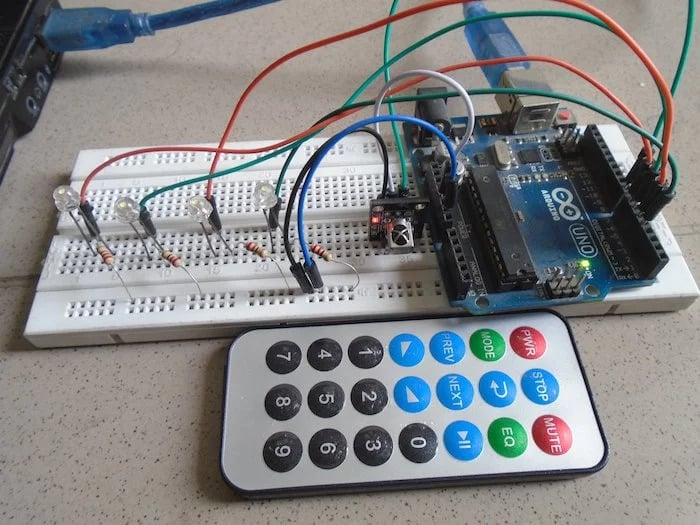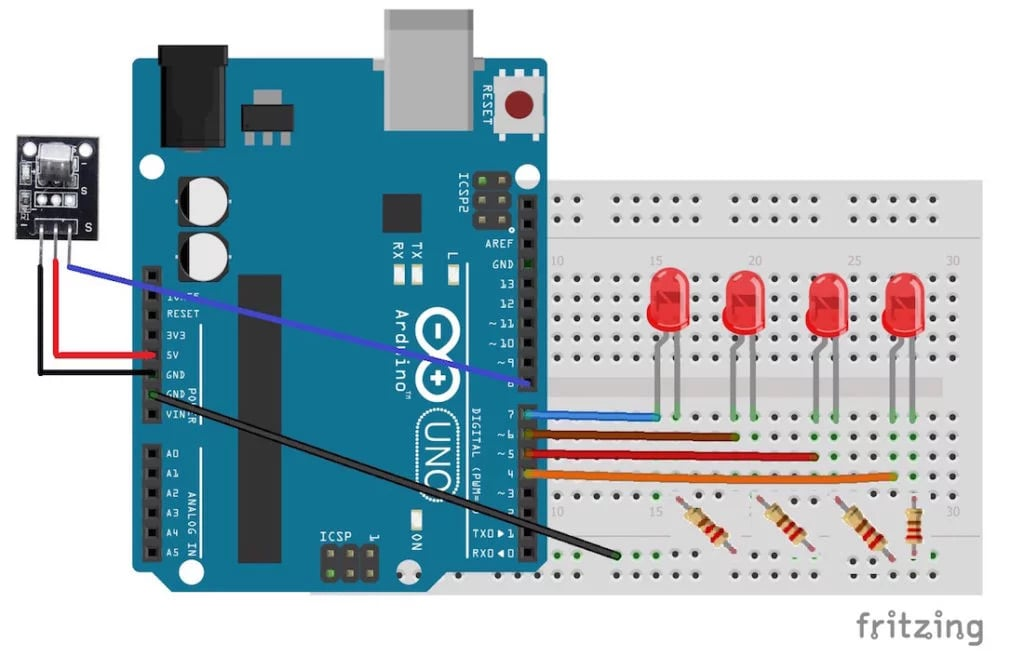Arduino

# How to Control LEDs With an Arduino, IR Sensor, and RemoteMarch 23, 2018 by A .
ShareUse an Arduino, infrared sensor, and remote to control LEDs.

In this project, we are going to control LEDs using an IR sensor and a remote. The IR sensor is a 1838B IR receiver. Whenever a button on the remote is pressed, it will send an infrared signal to the IR sensor in the coded form. The IR sensor will then receive this signal and will give it to the Arduino.### How Does It Work?

Whenever a button is pressed on the remote, it sends an infrared signal in encoded form. This signal is then received by the IR receiver and given to the Arduino.

We will save the code for the buttons that we want to control the LEDs in the Arduino code. Whenever a button on the remote is pressed, the Arduino receives a code. The Arduino will compare this code with the codes already saved, and if any of them match, the Arduino will turn on the LED connected to that button.

### Circuit Diagram

First, connect the four LEDs to the Arduino. Connect the positives of the four LEDs to the pins 7, 6, 5, and 4. Connect the negative of the four LEDs to GND on the Arduino through the 220 ohm resistors. The longer wires on the LEDs are positive and the shorter wires are negative.

Then connect the IR sensor to the Arduino. The connections for the IR sensor with the Arduino are as follows:

• Connect the negative wire on the IR sensor to GND on the Arduino.
• Connect the middle of the IR sensor which is the VCC to 5V on the Arduino.
• Connect the signal pin on the IR sensor to pin 8 on the Arduino.You can see a full-sized diagram here.

### Arduino Code

``````#include

#define first_key 48703
#define second_key 58359
#define third_key 539
#define fourth_key 25979

int first_led_pin = 7;
int second_led_pin = 6;
int third_led_pin = 5;
int fourth_led_pin = 4;
int led[] = {0,0,0,0};
decode_results output;

void setup()
{
Serial.begin(9600);
pinMode(first_led_pin, OUTPUT);
pinMode(second_led_pin, OUTPUT);
pinMode(third_led_pin, OUTPUT);
pinMode(fourth_led_pin, OUTPUT);
}

void loop() {
unsigned int value = output.value;
switch(value) {
case first_key:
if(led == 1) {
digitalWrite(first_led_pin, LOW);
led = 0;
} else {
digitalWrite(first_led_pin, HIGH);
led = 1;
}
break;
case second_key:

if(led == 1) {
digitalWrite(second_led_pin, LOW);
led = 0;
} else {
digitalWrite(second_led_pin, HIGH);
led = 1;
}
break;
case third_key:

if(led == 1) {
digitalWrite(third_led_pin, LOW);
led = 0;
} else {
digitalWrite(third_led_pin, HIGH);
led = 1;
}
break;
case fourth_key:

if(led == 1) {
digitalWrite(fourth_led_pin, LOW);
led = 0;
} else {
digitalWrite(fourth_led_pin, HIGH);
led = 1;
}
break;
}
Serial.println(value);
}
}``````

### Code Explanation

First of all, we added the library for the IR sensor and remote, then we defined the codes for the keys that we are going to use in our project. If you are using the code for the first time and do not know the code for the keys, then upload the Arduino code as it is and press the remote keysâ€”the code for the keys you pressed will be shown in the serial monitor. Now change this code with the previous code for the key that you want to control the LED with.

``````#include
#define first_key  48703
#define second_key  58359
#define third_key  539
#define fourth_key  25979

Next, we defined the pins where we have connected the LEDs. We have connected the LEDs at pins 7, 6, 5, and 4. So, we defined these pins as the LED pins.

``````int first_led_pin = 7;
int second_led_pin = 6;
int third_led_pin = 5;
int fourth_led_pin = 4;``````

In the setup function, we defined the LED pins as the output pins, because we are giving the output to the LEDs through those pins.

``````pinMode(first_led_pin, OUTPUT);
pinMode(second_led_pin, OUTPUT);
pinMode(third_led_pin, OUTPUT);
pinMode(fourth_led_pin, OUTPUT);``````

In the loop function, first, we check if any key has been pressed. If any key has been pressed, then we compare that key with the keys that we have defined in our code. If the key matches, then the LED connected to that pin will light up. If the LED connected to that pin is already lit up, then it will go down.

``````if (receiver.decode(&output)) {
unsigned int value = output.value;
switch(value) {
case first_key:
if(led == 1) {
digitalWrite(first_led_pin, LOW);
led = 0;
} else {
digitalWrite(first_led_pin, HIGH);
led = 1;
}
break;
case second_key:

if(led == 1) {
digitalWrite(second_led_pin, LOW);
led = 0;
} else {
digitalWrite(second_led_pin, HIGH);
led = 1;
}
break;
case third_key:

if(led == 1) {
digitalWrite(third_led_pin, LOW);
led = 0;
} else {
digitalWrite(third_led_pin, HIGH);
led = 1;
}
break;``````

### AuthorA .

For custom projects, hire me at https://www.freelancer.pk/u/Muhammadaqibdutt